Need Help?

Subscribe to Statics

###### \${selected_topic_name}
• Notes
• Comments & Questions

Determine the internal normal force, shear force. and moment at point $C$ .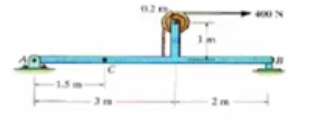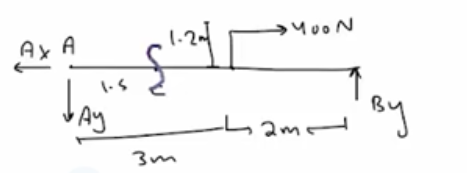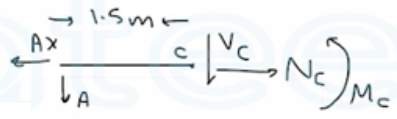$\sum M_{A}=0$

$B_ y(5)-400(1.2)=0$

$\rightarrow B_ y=96 N \uparrow$

$+\uparrow \sum F_ y=0$

$-A_ y+B _y=0 \rightarrow A_ y=96 N \downarrow$

$\stackrel{+}\rightarrow \sum F_{x}=0 \longrightarrow -A _x+400=0 \rightarrow A_ x=400 N \leftarrow$

$\stackrel{+}\rightarrow \sum F_{x}=0$

$N_{c}-400=0 \longrightarrow N_{c}=400 N \rightarrow$

$+\uparrow \sum F _y=0$

$-V_{c}-96=0 \longrightarrow V_{c}=96 N \uparrow$

$\sum M_C=0\quad\longrightarrow M_c+96(1.5)=0$

$∴ M_{c}=144 N \cdot m$

Determine the internal normal force, shear force,  and moment at points  $C$ and  $D$  in the simply supported  beam. Point  $D$  is located just to the left of the  $5 -kN$ force.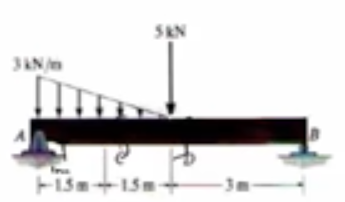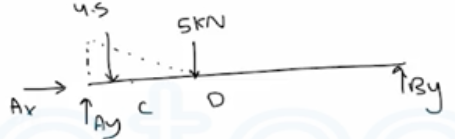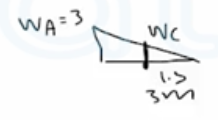$\frac{W_C}{1.5}=\frac{3}{3}$

$∴ W_{c}=1.5 \mathrm{kN} / \mathrm{m}$

$\sum M_{A}=0$

$B_ y(6)-5(3)-\frac{1}{2}(3)( 3 )(1)=0$

$∴ B_ y=3.25 k N$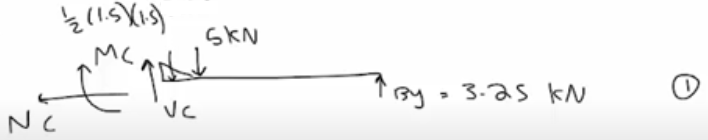Sam

$\stackrel{+}\rightarrow\sum F_{x}=0 \rightarrow N_{c}=0$

$+\uparrow\sum F_ y=0 \rightarrow V_{c}+3.25-\frac{1}{2}(1.5) (1.5 )-5=0$

$∴ V_{c}=2.875 k N$

$\sum M_{C}=0$

$3.25 (4.5)-\frac{1}{2}(1.5)(1.5)(0.5)-5(1.5)-M_c=0 \quad \longrightarrow M_c=6.56 kN.m$

Determine the internal normal force, shear force,  and moment at points  $C$  and  $D$  in the simply supported  beam. Point $D$  is located just to the left of the  $5-kN$ force.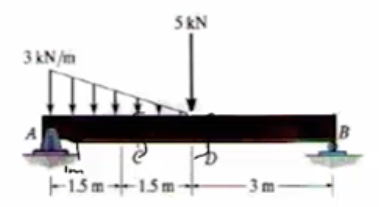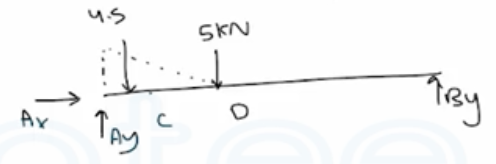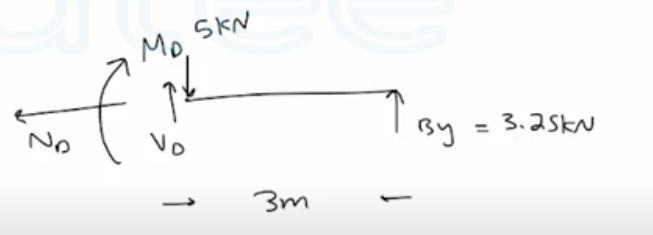$\stackrel{+}\longrightarrow \sum F_{x}=0 \quad \longrightarrow \quad N_{D}=0$

$+\uparrow \sum F_{y}=0$

$V_{D}+3.25-5=0$

$∴ V_{D}=1.75 k N$

$\sum M_{D}=0$

$3.25(3)-M_{D}=0$

$∴ M_{D}=9.75 k N . m$

No comments yet